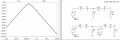# 4th Order Passive Resonant Circuit

#### Matjelo Naleli

Joined Oct 7, 2015
42
Suppose I have a series connection of resistor R, inductor L and a capacitor C forming a second order resonant circuit. A transfer function (say input voltage to capacitor voltage) of this circuit is that of a second order system with resonance frequency of (2*pi*L*C)^(-1/2) and quality factor of (R^2*C/L)^(-1/2).

My question
Is there a way of connecting two such circuits (or any 2nd order passive resonant circuits) such that the resulting transfer function (4th order) is the product of those of individual 2nd order resonant circuits both having the same frequency (but not necessarily the same quality factors)?

Another way of phrasing this question is to ask if one can built a 4th order passive resonant circuit whose transfer function can be decomposed into a product of two 2nd order transfer functions having the same resonant frequency.

The transfer function would look something like this:

G(s) = G1(s)*G2(s) = [N(s)/(s^2 + a1*s + b)]*[M(s)/(s^2 + a2*s + b)]

where s is the Laplace parameter and N(s) and M(s) can be any polynomials of s with order not greater than 2.

Thanks

#### Veracohr

Joined Jan 3, 2011
771
Another way of phrasing this question is to ask if one can built a 4th order passive resonant circuit whose transfer function can be decomposed into a product of two 2nd order transfer functions having the same resonant frequency.
In general, yes. If you make the impedances of the second section high enough relative to the first section, the loading will be minimal and a simple product of the two transfer functions describes the circuit.

As an example, here you see two 4th order circuits, where the second one has the two sections separated by a perfect buffer (voltage controlled voltage source) to eliminate loading effects. In this case the output (V2) is the product of two identical 2nd order circuits. The AC responses of the buffered and non-buffered circuits are within 0.5dB of each other, deviating around the peak. Notice that the components in the second section of the top circuit are scaled by a factor of 10 - if you make the components exactly equal in the top circuit the response around the peak deviates by up to 3.5dB because of loading.#### Matjelo Naleli

Joined Oct 7, 2015
42
Exellent response Veracohr and straight to the point. This minimal loading seems to be the key trick in this as I see it now. I will follow your examples and see how I can achieve the minimal loading with the last stage output voltage measured across the capacitor (or resistor plus capacitor). I am actually aiming to use this resonator for our RF linear Paul trap (ion trap) [at 15MHz] in the lab which can essentially be modelled as capacitor with a small parasitic resistivity.

If I may ask, what is the name of the software you used for simulating these circuits?

Last edited:

#### Veracohr

Joined Jan 3, 2011
771

#### Matjelo Naleli

Joined Oct 7, 2015
42
Ohh, I am familiar with it displaying bode plots with black background. Anyway I haven't played around with it that much.

Thanks Veracohr.

#### Veracohr

Joined Jan 3, 2011
771

#### Matjelo Naleli

Joined Oct 7, 2015
42
I am now able to increase the quality factor of my compound resonator by just cascading several second order resonators in a ladder pattern as you showed me earlier with two stages. Also my plots are white now so I am happy, thanks to you Veracohr.

Now I want to compare the AC response of my ladder circuit of cascaded resonators to its buffered equivalent as you did in your circuits examples, however I am not sure where to get the buffer that you used in your second circuit. Did you build it yourself or is it a built-in component in LTSpice? If it's built-in where can I get it?

Thanks

#### Veracohr

Joined Jan 3, 2011
771
It's a basic component, called Voltage Dependent Voltage Source, with the symbol e.

#### Matjelo Naleli

Joined Oct 7, 2015
42
Ohh I got it, sorry. Thank you.

#### ramancini8

Joined Jul 18, 2012
473
I hope this is just a paper exercise because some real problems will occur when you build it.